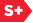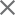Share Email Print### Proceedings Paper

Multiresolution estimation of motion using the wavelet transform
Author(s): Enrique Barria; Jogikal M. Jagadeesh
Format Member Price Non-Member Price
PDF \$17.00 \$21.00

Paper Abstract

Many applications in research, in manufacturing and in every day life require measurement of velocity. Generally the velocity is estimated from a sequence of images. There are many methods reported in the literature using heuristics and transform techniques. In general these methods are tailored to specific applications. We propose a wavelet transform based technique to estimate velocity. We assume that the scene can be piecewise modeled by a multi variable polynomial. We have derived wavelet functions that can be used to estimate velocity from components of the wavelet transform. The technique has been demonstrated for 1D analytical functions and the error is less than 0.5% for polynomials of finite degree, and less than 5% for sinusoids. For 2D sequence of images, the image space is represented by a spatio temporal cube, and partitioned into subcubes of different dimensions. Wavelet transform techniques can then be applied to these variable size segments at multiple resolution. To estimate the two velocity components Vx and Vy in this spatio temporal cube requires the solution of a system of 8 non linear equations. We are in the process of solving these non linear equations.

Paper Details

Date Published: 11 October 1994
PDF: 12 pages
Proc. SPIE 2303, Wavelet Applications in Signal and Image Processing II, (11 October 1994); doi: 10.1117/12.188803
Show Author Affiliations
Enrique Barria, The Ohio State Univ. (United States)
Jogikal M. Jagadeesh, The Ohio State Univ. (United States)

Published in SPIE Proceedings Vol. 2303:
Wavelet Applications in Signal and Image Processing II
Andrew F. Laine; Michael A. Unser, Editor(s)